# Clustering

### Comparing Cluster Algorithms on Density Data

Posted on Updated on

In a previous posted I gave a detailed description of using DBScan to create clusters for a dataset containing different density based data. This “manufactured” dataset was created to illustrate how and why DBScan can be used.

But taking the previous post in isolation is perhaps not recommended. As a Data Scientist you will need to use many Clustering algorithms to determine which algorithm can best identify the patterns in your data, and this can be determined by the type of data distributions within the dataset.

The DBScan post created the following diagrams. The diagram on the left is a plot of the dataset where we can easily identify different groupings/clusters. The diagram on the right illustrates the clusters identified by DBScan. As you can see it did a good job.

We can see the three clusters and the noisy data point which were added to the dataset.

But what about other Clustering algorithms? What about k-Means and Hierarchical Clustering algorithms? How would they perform on this dataset?

Here is the code for k-Means with three clusters. Three clusters was selected as we have three clear clusters in the dataset.

```#k-Means with 3 clusters
from sklearn.cluster import KMeans
k_means=KMeans(n_clusters=3,random_state=42)
k_means.fit(df[[0,1]])

df['KMeans_labels']=k_means.labels_

# Plotting resulting clusters
colors=['purple','red','blue','green']
plt.figure(figsize=(10,10))
plt.scatter(df,df,c=df['KMeans_labels'],cmap=matplotlib.colors.ListedColormap(colors),s=15)
plt.title('K-Means Clustering',fontsize=18)
plt.xlabel('Feature-1',fontsize=12)
plt.ylabel('Feature-2',fontsize=12)
plt.show()```

Here is the code for Hierarchical Clustering, again three clusters was selected.

```from sklearn.cluster import AgglomerativeClustering
model = AgglomerativeClustering(n_clusters=3, affinity='euclidean')
model.fit(df[[0,1]])

df['HR_labels']=model.labels_

# Plotting resulting clusters
plt.figure(figsize=(10,10))
plt.scatter(df,df,c=df['HR_labels'],cmap=matplotlib.colors.ListedColormap(colors),s=15)
plt.title('Hierarchical Clustering',fontsize=20)
plt.xlabel('Feature-1',fontsize=14)
plt.ylabel('Feature-2',fontsize=14)
plt.show()```

The diagrams from both of these are shown below.

As you can see the results generated by these alternative Clustering algorithms produce very different results to what was produced by DBScan (see image at top of post) and we can easily see which algorithm best fits the dataset used.

Make sure you check out the post on DBScan.

### DBScan Clustering in Python

Posted on Updated on

Unsupervised Learning is a common approach for discovering patterns in datasets. The main algorithmic approach in Unsupervised Learning is Clustering, where the data is searched to discover groupings, or clusters, of data. Each of these clusters contain data points which have some set of characteristics in common with each other, and each cluster is distinct and different. There are many challenges with clustering which include trying to interpret the meaning of each cluster and how it is related to the domain in question, what is the “best” number of clusters to use or have, the shape of each cluster can be different (not like the nice clean examples we see in the text books), clusters can be overlapping with a data point belonging to many different clusters, and the difficulty with trying to decide which clustering algorithm to use.

The last point above about which clustering algorithm to use is similar to most problems in Data Science and Machine Learning. The simple answer is we just don’t know, and this is where the phases of “No free lunch” and “All models are wrong, but some models are model that others”, apply. This is where we need to apply the various algorithms to our data, and through a deep process of investigation the outputs, of each algorithm, need to be investigated to determine what algorithm, the parameters, etc work best for our dataset, specific problem being investigated and the domain. This involve the needs for lots of experiments and analysis. This work can take some/a lot of time to complete.

The k-Means clustering algorithm gets a lot of attention and focus for Clustering. It’s easy to understand what it does and to interpret the outputs. But it isn’t perfect and may not describe your data, as it can have different characteristics including shape, densities, sparseness, etc. k-Means focuses on a distance measure, while algorithms like DBScan can look at the relative densities of data. These two different approaches can produce by different results. Careful analysis of the data and the results/outcomes of these algorithms needs some care.

Let’s illustrate the use of DBScan (Density Based Spatial Clustering of Applications with Noise), using the scikit-learn Python package, for a “manufactured” dataset. This example will illustrate how this density based algorithm works (See my other blog post which compares different Clustering algorithms for this same dataset). DBSCAN is better suited for datasets that have disproportional cluster sizes (or densities), and whose data can be separated in a non-linear fashion.

There are two key parameters of DBScan:

• eps: The distance that specifies the neighborhoods. Two points are considered to be neighbors if the distance between them are less than or equal to eps.
• minPts: Minimum number of data points to define a cluster.

Based on these two parameters, points are classified as core point, border point, or outlier:

• Core point: A point is a core point if there are at least minPts number of points (including the point itself) in its surrounding area with radius eps.
• Border point: A point is a border point if it is reachable from a core point and there are less than minPts number of points within its surrounding area.
• Outlier: A point is an outlier if it is not a core point and not reachable from any core points.

The algorithm works by randomly selecting a starting point and it’s neighborhood area is determined using radius eps. If there are at least minPts number of points in the neighborhood, the point is marked as core point and a cluster formation starts. If not, the point is marked as noise. Once a cluster formation starts (let’s say cluster A), all the points within the neighborhood of initial point become a part of cluster A. If these new points are also core points, the points that are in the neighborhood of them are also added to cluster A. Next step is to randomly choose another point among the points that have not been visited in the previous steps. Then same procedure applies. This process finishes when all points are visited.

Let’s setup our data set and visualize it.

```import numpy as np
import pandas as pd
import math
import matplotlib.pyplot as plt
import matplotlib

#initialize the random seed
np.random.seed(42) #it is the answer to everything!

#Create a function to create our data points in a circular format
#We will call this function below, to create our dataframe
def CreateDataPoints(r, n):
return [(math.cos(2*math.pi/n*x)*r+np.random.normal(-30,30),math.sin(2*math.pi/n*x)*r+np.random.normal(-30,30)) for x in range(1,n+1)]

#Use the function to create different sets of data, each having a circular format
df=pd.DataFrame(CreateDataPoints(800,1500))  #500, 1000
df=df.append(CreateDataPoints(500,850))      #300, 700
df=df.append(CreateDataPoints(200,450))      #100, 300

# Adding noise to the dataset
df=df.append([(np.random.randint(-850,850),np.random.randint(-850,850)) for i in range(450)])

plt.figure(figsize=(8,8))
plt.scatter(df,df,s=15,color='olive')
plt.title('Dataset for DBScan Clustering',fontsize=16)
plt.xlabel('Feature-1',fontsize=12)
plt.ylabel('Feature-2',fontsize=12)
plt.show()```

We can see the dataset we’ve just created has three distinct circular patterns of data. We also added some noisy data too, which can be see as the points between and outside of the circular patterns.

Let’s use the DBScan algorithm, using the default setting, to see what it discovers.

```from sklearn.cluster import DBSCAN
#DBSCAN without any parameter optimization and see the results.
dbscan=DBSCAN()
dbscan.fit(df[[0,1]])

df['DBSCAN_labels']=dbscan.labels_

# Plotting resulting clusters
colors=['purple','red','blue','green']
plt.figure(figsize=(8,8))
plt.scatter(df,df,c=df['DBSCAN_labels'],cmap=matplotlib.colors.ListedColormap(colors),s=15)
plt.title('DBSCAN Clustering',fontsize=16)
plt.xlabel('Feature-1',fontsize=12)
plt.ylabel('Feature-2',fontsize=12)
plt.show()
#Not very useful !
#Everything belongs to one cluster. ```

Everything is the one color! which means all data points below to the same cluster. This isn’t very useful and can at first seem like this algorithm doesn’t work for our dataset. But we know it should work given the visual representation of the data. The reason for this occurrence is because the value for epsilon is very small. We need to explore a better value for this. One approach is to use KNN (K-Nearest Neighbors) to calculate the k-distance for the data points and based on this graph we can determine a possible value for epsilon.

```#Let's explore the data and work out a better setting
from sklearn.neighbors import NearestNeighbors
neigh = NearestNeighbors(n_neighbors=2)
nbrs = neigh.fit(df[[0,1]])
distances, indices = nbrs.kneighbors(df[[0,1]])

# Plotting K-distance Graph
distances = np.sort(distances, axis=0)
distances = distances[:,1]
plt.figure(figsize=(14,8))
plt.plot(distances)
plt.title('K-Distance - Check where it bends',fontsize=16)
plt.xlabel('Data Points - sorted by Distance',fontsize=12)
plt.ylabel('Epsilon',fontsize=12)
plt.show()
#Let’s plot our K-distance graph and find the value of epsilon```

Look at the graph above we can see the main curvature is between 20 and 40. Taking 30 at the mid-point of this we can now use this value for epsilon. The value for the number of samples needs some experimentation to see what gives the best fit.

Let’s now run DBScan to see what we get now.

```from sklearn.cluster import DBSCAN
dbscan_opt=DBSCAN(eps=30,min_samples=3)
dbscan_opt.fit(df[[0,1]])

df['DBSCAN_opt_labels']=dbscan_opt.labels_
df['DBSCAN_opt_labels'].value_counts()

# Plotting the resulting clusters
colors=['purple','red','blue','green', 'olive', 'pink', 'cyan', 'orange', 'brown' ]
plt.figure(figsize=(8,8))
plt.scatter(df,df,c=df['DBSCAN_opt_labels'],cmap=matplotlib.colors.ListedColormap(colors),s=15)
plt.title('DBScan Clustering',fontsize=18)
plt.xlabel('Feature-1',fontsize=12)
plt.ylabel('Feature-2',fontsize=12)
plt.show()```

When we look at the dataframe we can see it create many different cluster, beyond the three that we might have been expecting. Most of these clusters contain small numbers of data points. These could be considered outliers and alternative view of this results is presented below, with this removed.

```df['DBSCAN_opt_labels']=dbscan_opt.labels_
df['DBSCAN_opt_labels'].value_counts()

0     1559
2      898
3      470
-1      282
8        6
5        5
4        4
10       4
11       4
6        3
12       3
1        3
7        3
9        3
13       3
Name: DBSCAN_opt_labels, dtype: int64```

The cluster labeled with -1 contains the outliers. Let’s clean this up a little.

```df2 = df[df['DBSCAN_opt_labels'].isin([-1,0,2,3])]
df2['DBSCAN_opt_labels'].value_counts()
0    1559
2     898
3     470
-1     282
Name: DBSCAN_opt_labels, dtype: int64

# Plotting the resulting clusters
colors=['purple','red','blue','green', 'olive', 'pink', 'cyan', 'orange']
plt.figure(figsize=(8,8))
plt.scatter(df2,df2,c=df2['DBSCAN_opt_labels'],cmap=matplotlib.colors.ListedColormap(colors),s=15)
plt.title('DBScan Clustering',fontsize=18)
plt.xlabel('Feature-1',fontsize=12)
plt.ylabel('Feature-2',fontsize=12)
plt.show()```

See my other blog post which compares different Clustering algorithms for this same dataset.

### Cluster Distance using SQL with Oracle Data Mining – Part 4

Posted on Updated on

This is the fourth and last blog post in a series that looks at how you can examine the details of predicted clusters using Oracle Data Mining. In the previous blog posts I looked at how to use CLUSER_ID, CLUSTER_PROBABILITY and CLUSTER_SET.

In this blog post we will look at CLUSTER_DISTANCE. We can use the function to determine how close a record is to the centroid of the cluster. Perhaps we can use this to determine what customers etc we might want to focus on most. The customers who are closest to the centroid are one we want to focus on first. So we can use it as a way to prioritise our workflows, particularly when it is used in combination with the value for CLUSTER_PROBABILITY.

Here is an example of using CLUSTER_DISTANCE to list all the records that belong to Cluster 14 and the results are ordered based on closeness to the centroid of this cluster.

```SELECT customer_id,
cluster_probability(clus_km_1_37 USING *) as cluster_Prob,
cluster_distance(clus_km_1_37 USING *) as cluster_Distance
FROM   insur_cust_ltv_sample
WHERE   cluster_id(clus_km_1_37 USING *) = 14
order by cluster_Distance asc;
```

Here is a subset of the results from this query.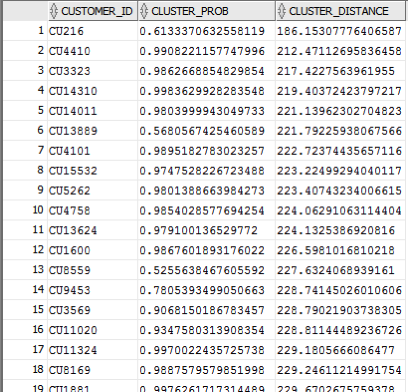When you examine the results you may notice that the records that is listed first and closest record to the centre of cluster 14 has a very low probability. You need to remember that we are working in a N-dimensional space here. Although this first record is closest to the centre of cluster 14 it has a really low probability and if we examine this record in more detail we will find that it is at an overlapping point between a number of clusters.

This is why we need to use the CLUSTER_DISTANCE and CLUSTER_PROBABILITY functions together in our workflows and applications to determine how we need to process records like these.

### Cluster Sets using SQL with Oracle Data Mining – Part 3

Posted on Updated on

This is the third blog post on my series on examining the Clusters that were predicted by an Oracle Data Mining model. Check out the previous blog posts.

In the previous posts we were able to list the predicted cluster for each record in our data set. This is the cluster that the records belonged to the most. I also mentioned that a record could belong to many clusters.

So how can you list all the clusters that the a record belongs to?

You can use the CLUSTER_SET SQL function. This will list the Cluster Id and a probability measure for each cluster. This function returns a array consisting of the set of all clusters that the record belongs to.

The following example illustrates how to use the CLUSTER_SET function for a particular cluster model.

```SELECT t.customer_id, s.cluster_id, s.probability
FROM   (select customer_id, cluster_set(clus_km_1_37 USING *) as Cluster_Set
from   insur_cust_ltv_sample
WHERE  customer_id in ('CU13386', 'CU100')) T,
TABLE(T.cluster_set) S
order by t.customer_id, s.probability desc;
```

The output from this query will be an ordered data set based on the customer id and then the clusters listed in descending order of probability. The cluster with the highest probability is what would be returned by the CLUSTER_ID function. The output from the above query is shown below.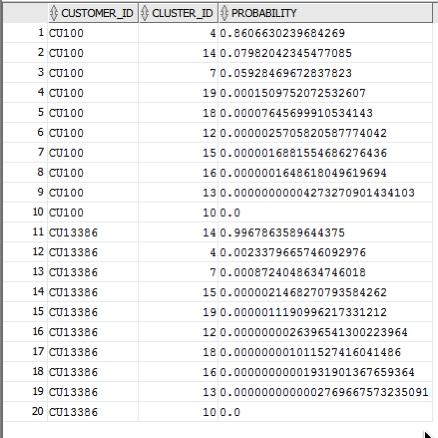If you would like to see the details of each of the clusters and to examine the differences between these clusters then you will need to use the CLUSTER_DETAILS function (see previous blog post).

You can specify topN and cutoff to limit the number of clusters returned by the function. By default, both topN and cutoff are null and all clusters are returned.

– topN is the N most probable clusters. If multiple clusters share the Nth probability, then the function chooses one of them.

– cutoff is a probability threshold. Only clusters with probability greater than or equal to cutoff are returned. To filter by cutoff only, specify NULL for topN.

You may want to use these individually or combined together if you have a large number of customers. To return up to the N most probable clusters that are greater than or equal to cutoff, specify both topN and cutoff.

The following example illustrates using the topN value to return the top 4 clusters.

```SELECT t.customer_id, s.cluster_id, s.probability
FROM   (select customer_id, cluster_set(clus_km_1_37, 4, null USING *) as Cluster_Set
from   insur_cust_ltv_sample
WHERE  customer_id in ('CU13386', 'CU100')) T,
TABLE(T.cluster_set) S
order by t.customer_id, s.probability desc;
```

and the output from this query shows only 4 clusters displayed for each record.Alternatively you can select the clusters based on a cut off value for the probability. In the following example this is set to 0.05.

```SELECT t.customer_id, s.cluster_id, s.probability
FROM   (select customer_id, cluster_set(clus_km_1_37, NULL, 0.05 USING *) as Cluster_Set
from   insur_cust_ltv_sample
WHERE  customer_id in ('CU13386', 'CU100')) T,
TABLE(T.cluster_set) S
order by t.customer_id, s.probability desc;
```

and the output this time looks a bit different.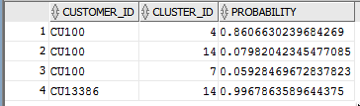Finally, yes you can combine these two parameters to work together.

SELECT t.customer_id, s.cluster_id, s.probability
FROM (select customer_id, cluster_set(clus_km_1_37, 2, 0.05 USING *) as Cluster_Set
from insur_cust_ltv_sample
WHERE customer_id in (‘CU13386’, ‘CU100’)) T,
TABLE(T.cluster_set) S
order by t.customer_id, s.probability desc;

### Examining predicted Clusters and Cluster details using SQL

Posted on

In a previous blog post I gave some details of how you can examine some of the details behind a prediction made using a classification model. This seemed to spark a lot of interest. But before I come back to looking at classification prediction details and other information, this blog post is the first in a 4 part blog post on examining the details of Clusters, as identified by a cluster model created using Oracle Data Mining.

The 4 blog posts will consist of:

• 1 – (this blog post) will look at how to determine the predicted cluster and cluster probability for your record.
• 2 – will show you how to examine the details behind and used to predict the cluster.
• 3 – A record could belong to many clusters. In this blog post we will look at how you can determine what clusters a record can belong to.
• 4 – Cluster distance is a measure of how far the record is from the cluster centroid. As a data point or record can belong to many clusters, it can be useful to know the distances as you can build logic to perform different actions based on the cluster distances and cluster probabilities.

Right. Let’s have a look at the first set of these closer functions. These are CLUSTER_ID and CLUSTER_PROBABILITY.

CLUSER_ID : Returns the number of the cluster that the record most closely belongs to. This is measured by the cluster distance to the centroid of the cluster. A data point or record can belong or be part of many clusters. So the CLUSTER_ID is the cluster number that the data point or record most closely belongs too.

CLUSTER_PROBABILITY : Is a probability measure of the likelihood of the data point or record belongs to a cluster. The cluster with the highest probability score is the cluster that is returned by the CLUSTER_ID function.

Now let us have a quick look at the SQL for these two functions. This first query returns the cluster number that each record most strong belongs too.

```SELECT customer_id,
cluster_id(clus_km_1_37 USING *) as Cluster_Id,
FROM   insur_cust_ltv_sample
WHERE  customer_id in ('CU13386', 'CU6607', 'CU100');
```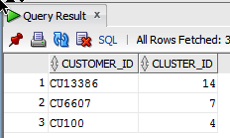Now let us add in the cluster probability function.

```SELECT customer_id,
cluster_id(clus_km_1_37 USING *) as Cluster_Id,
cluster_probability(clus_km_1_37 USING *) as cluster_Prob
FROM   insur_cust_ltv_sample
WHERE  customer_id in ('CU13386', 'CU6607', 'CU100');
```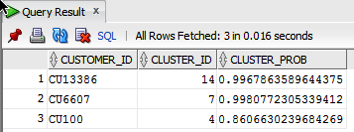These functions gives us some insights into what the cluster predictive model is doing. In the remaining blog posts in this series I will look at how you can delve deeper into the predictions that the cluster algorithm is make.